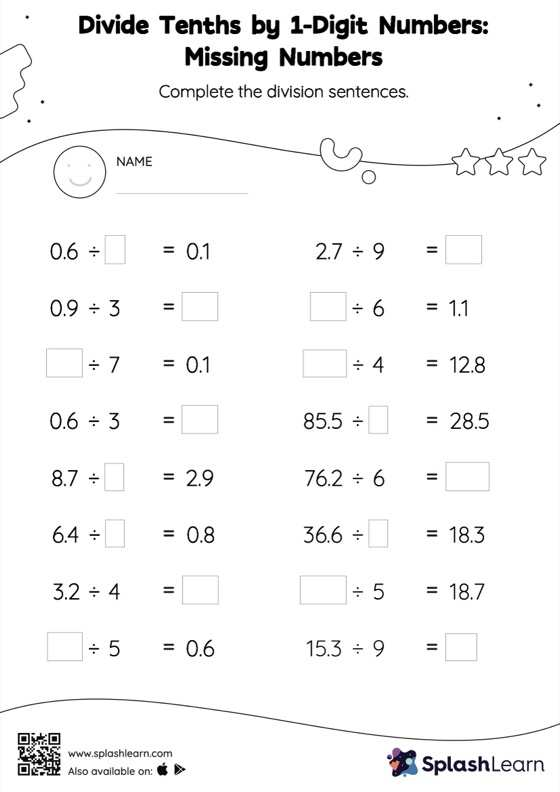# Divide Tenths by 1-Digit Numbers without Remainder: Missing Numbers Worksheet

Home > Divide Tenths by 1-Digit Numbers without Remainder: Missing NumbersTask your little mathematicians to crack the code of dividing tenths by 1-digit numbers without remainder with this fun worksheet. Students divide decimals by 1-digit numbers in the same manner as they would divide whole numbers first. Depending on how many decimal digits are in the dividend, they add a decimal point at the appropriate place in the quotient. They apply this concept to find the missing number in divide tenths by 1-digit numbers without remainder worksheet. In each problem, the numbers are laid out in the horizontal format. Students should try to use different strategies involving composing and decomposing numbers to solve these problems. This will help them develop flexibility and fluency.